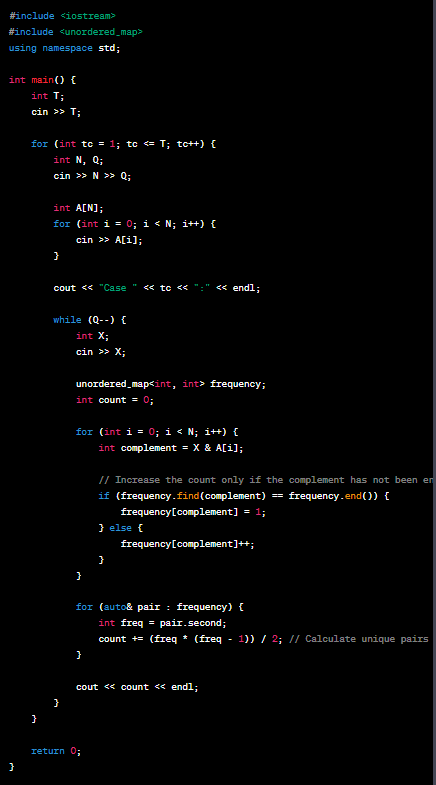# Aliban Vs AIFellow_juniorDIU Take-Off Programming...5/8/15
Limits 2s, 32 MB

Uban and Aliban are $2^{nd}$ year undergraduate students. Uban is a newbie sport programmer, whereas Aliban is blue. Recently they came to know about FatGpt. FatGpt is a chatbot software that simulates human-like conversations with users via chat. Its essential task is to answer user questions with instant messages. After hearing about Chatbot AI, Uban, and Aliban argue that AI can solve any problem. Uban thinks AI can replace humans, whereas Aliban says AI can only solve easy to medium-level tasks and can't solve critical thinking tasks. Their argument finished without any final statement.

After a few days back, Aliban finds an interesting problem in an online judge, which needs complex thinking to solve. Aliban comes to Uban and says, "I find an interesting problem, and I can solve this. Let's challenge FatGpt, and I am sure it will prove you wrong that AI can replace humans."

Uban tells FatGpt about the problem statement and asks to write a C++ solution. The conversation between Uban and FatGpt is given below:

Uban's Question: "You are given an array $A$ of length $N$. You have to process $Q$ queries. A single integer $X$ represents each query. In each query, you have to find the number of unique pairs such that$A_i \:\&\: A_j = X$ . Here, $\&$ means bitwise and operator.”

FatGpt's Response:After getting FatGpt's response, Uban became excited and started yelling at Aliban, “You See….”. Aliban says, "Let's submit it and make you surprised." After submitting FatGpt's solution, they got “Wrong Answer”.

Now your task is to write the correct solution to this problem. The definition of the unique pairs can be found in the note section.

## Input

The first line contains a positive number $T$ $(1 \le T \le 10)$ — the number of test cases. The following is a description of the test cases.

The first line of the description of each test case contains two positive numbers $N$ $(1 \le N \le 2*10^{5})$ and $Q$ $(1 \le Q \le 10^{5})$ — the size of the array and the number of queries.

The second line of the description of each test case contains $N$ integers $A_1,A_2,…,A_N$ $(0 \le A_i \le 10^{6})$ — the elements of array $A$.

Then $Q$ lines follow; each line contains a number $X$ $(0 \le X \le 10^{6})$, giving the queries.

## Output

For each test case, print “Case $tc$:”, where $tc$ means the number of the test case. After that, for each query, print the number of unique pairs. See the sample test case for a better understanding of the output format.

## Sample

InputOutput
2
7 3
78 1 34 33 1 14 89
4
1
3
9 4
1 2 3 4 5 6 7 8 9
2
3
9
1

Case 1:
0
4
0
Case 2:
4
1
0
8


Unique pairs: Let's say an array has elements [1, 2, 4, 5, 1]. Then there will be $7$ unique pairs - $(1,2), (1,4), (1,5), (1,1), (2,4), (2,5), (4,5)$. $(1,2)$ and $(2,1)$is considered same.

### Submit